# Finding values to make a linear system consistent

## Homework Statement

Given the following matrix: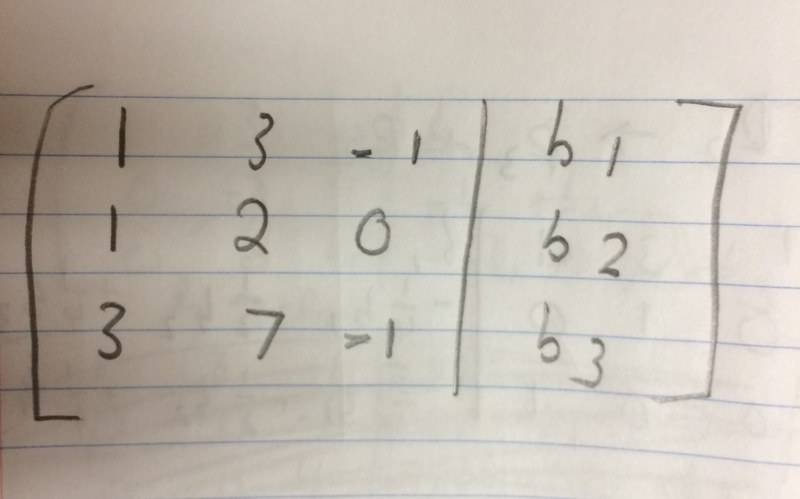I need to determine the conditions for b1, b2, and b3 to make the system consistent. In addition, I need to check if the system is consistent when:
a) b1 = 1, b2 = 1, b3 = 3
b) b1 = 1, b2 = 0., b3 = -1
c) b1 = 1, b2 = 2, b3 = 3

## Homework Equations

Gaussian elimination method I used here:
http://mathworld.wolfram.com/GaussianElimination.html

## The Attempt at a Solution

For the matrix to be consistent, I knew that the number of non-zero rows had to be less than the number of columns. Hence I tried to get the last row to be 0 0 0 | *; however while I managed to get the last row to become 0 0 1, I don't know how to make it the zero row I want.

I'm wondering if my thought process to make this consistent is correct, or if there is another way I can make this system consistent.

#### Attachments

Homework Helper
Gold Member
Deduct multiples of the first row from the second and third rows, where the multipliers used are chosen to make the first element of the modified second and third rows be zero.

From there it is easy to make the last row zero (in the first three columns). If it doesn't look easy, post what you get on here.

Deduct multiples of the first row from the second and third rows, where the multipliers used are chosen to make the first element of the modified second and third rows be zero.

From there it is easy to make the last row zero (in the first three columns). If it doesn't look easy, post what you get on here.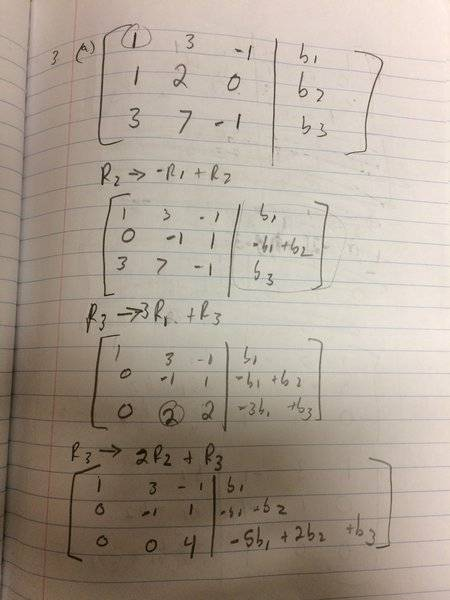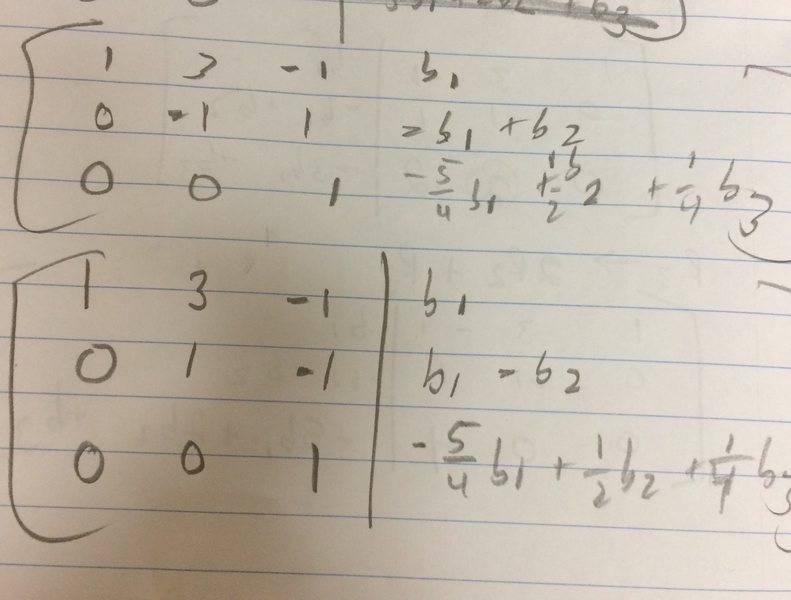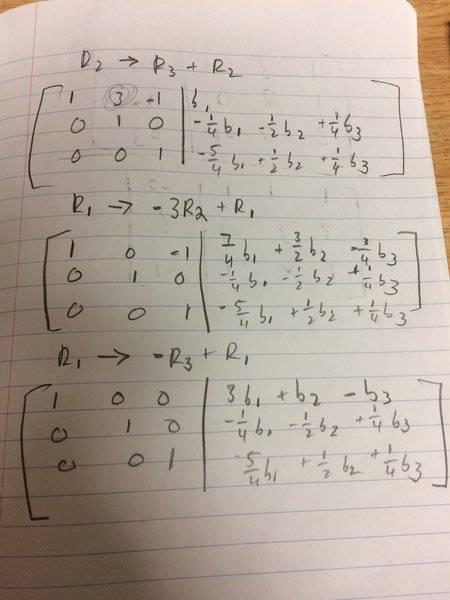This is what I got from simplifying my matrix. But since the rows all have zeroes at different columns, how can I reduce one of the rows to 0 0 0?

#### Attachments

Homework Helper
Gold Member
There's an arithmetic error. You have got a sign wrong in the operation you perform on row 3. Fix that and it should all fall into place.

There's an arithmetic error. You have got a sign wrong in the operation you perform on row 3. Fix that and it should all fall into place.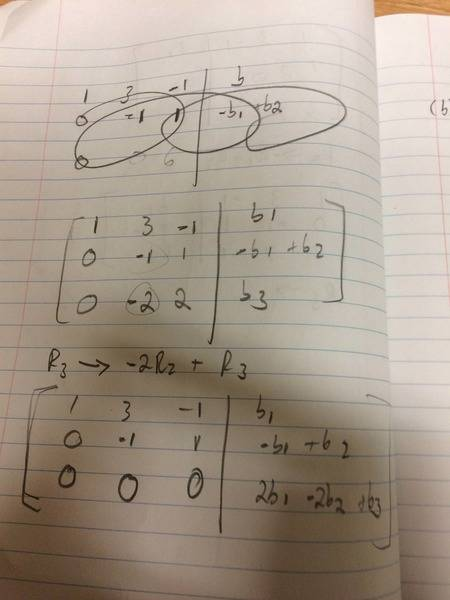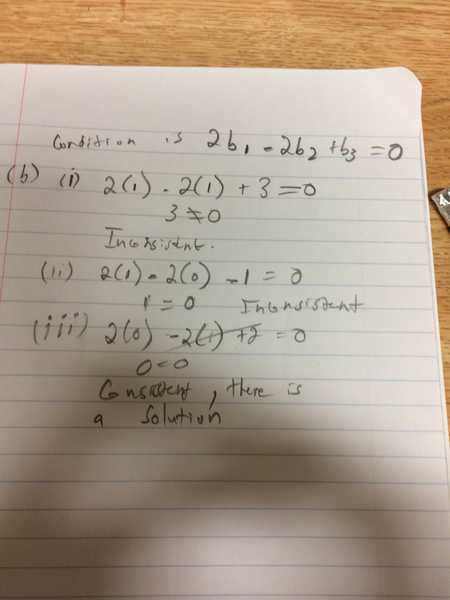This is the method I used to find out how the system could be consistent. Is my process for determining the condition and testing it for the given values the correct method?